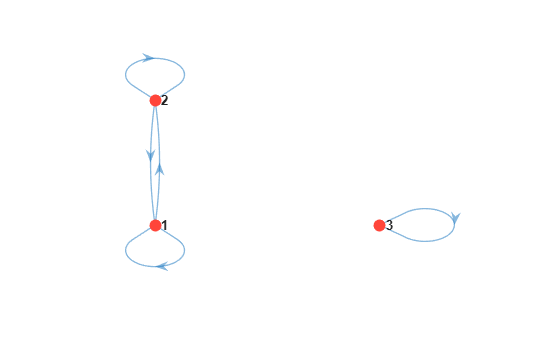# isreducible

Check Markov chain for reducibility

## Syntax

``tf = isreducible(mc)``

## Description

example

``tf = isreducible(mc)` returns `true` if the discrete-time Markov chain `mc` is reducible and `false` otherwise.`

## Examples

collapse all

Consider this three-state transition matrix.

`$P=\left[\begin{array}{ccc}0.5& 0.5& 0\\ 0.5& 0.5& 0\\ 0& 0& 1\end{array}\right]$`

Create the Markov chain that is characterized by the transition matrix P.

```P = [0.5 0.5 0; 0.5 0.5 0; 0 0 1]; mc = dtmc(P);```

Determine whether the Markov chain is reducible.

`isreducible(mc)`
```ans = logical 1 ```

`1` indicates that `mc` is reducible.

Visually confirm the reducibility of the Markov chain by plotting its digraph.

```figure; graphplot(mc);```Two independent chains appear in the figure. This result indicates that you can analyze the two chains separately.

## Input Arguments

collapse all

Discrete-time Markov chain with `NumStates` states and transition matrix `P`, specified as a `dtmc` object. `P` must be fully specified (no `NaN` entries).

## Output Arguments

collapse all

Reducibility flag, returned as `true` if `mc` is a reducible Markov chain and `false` otherwise.

collapse all

### Reducible Chain

A Markov chain is reducible if it consists of more than one communicating class. Asymptotic analysis is reduced to individual subclasses. See `classify` and `asymptotics`.

## Algorithms

• The Markov chain `mc` is irreducible if every state is reachable from every other state in at most n – 1 steps, where n is the number of states (`mc.NumStates`). This result is equivalent to Q = (I + Z)n – 1 containing all positive elements. I is the n-by-n identity matrix. The zero-pattern matrix of the transition matrix P (`mc.P`) is Zij = I(Pij > 0), for all i,j . To determine reducibility, `isreducible` computes Q.

• By the Perron-Frobenius Theorem , irreducible Markov chains have unique stationary distributions. Unichains, which consist of a single recurrent class plus transient classes, also have unique stationary distributions (with zero probability mass in the transient classes). Reducible chains with multiple recurrent classes have stationary distributions that depend on the initial distribution.

 Gallager, R.G. Stochastic Processes: Theory for Applications. Cambridge, UK: Cambridge University Press, 2013.

 Horn, R., and C. R. Johnson. Matrix Analysis. Cambridge, UK: Cambridge University Press, 1985.